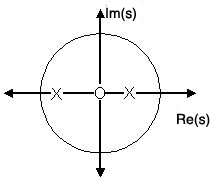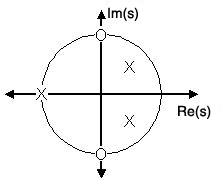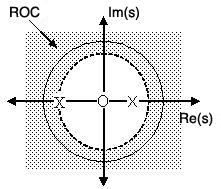# 11.4 Poles and zeros in the s-plane

 Page 1 / 1
This module will look at the relationships between the Laplace transform and the complex plane. Specifically, the creation of pole/zero plots and some of their useful properties are discussed.

## Introduction to poles and zeros of the laplace-transform

It is quite difficult to qualitatively analyze the Laplace transform and Z-transform , since mappings of their magnitude and phase or real part andimaginary part result in multiple mappings of 2-dimensional surfaces in 3-dimensional space. For this reason, it is verycommon to examine a plot of a transfer function's poles and zeros to try to gain a qualitative idea of what a system does.

Once the Laplace-transform of a system has been determined, one can use the information contained in function's polynomials tographically represent the function and easily observe many defining characteristics. The Laplace-transform will have the belowstructure, based on Rational Functions :

$H(s)=\frac{P(s)}{Q(s)}$

The two polynomials, $P(s)$ and $Q(s)$ , allow us to find the poles and zeros of the Laplace-Transform.

zeros
The complex frequencies that make the overall gain of the filter transfer function zero.
poles
The complex frequencies that make the overall gain of the filter transfer function infinite.

Below is a simple transfer function with the poles and zeros shown below it. $H(s)=\frac{s+1}{(s-\frac{1}{2})(s+\frac{3}{4})}$

The zeros are: $\{-1\}$

The poles are: $\{\frac{1}{2}, -\left(\frac{3}{4}\right)\}$

## The s-plane

Once the poles and zeros have been found for a given Laplace Transform, they can be plotted onto the S-Plane. TheS-plane is a complex plane with an imaginary and real axis referring to the complex-valued variable $z$ . The position on the complex plane is given by $re^{i\theta }$ and the angle from the positive, real axis around the plane is denoted by $\theta$ . When mapping poles and zeros onto the plane, poles are denoted byan "x" and zeros by an "o". The below figure shows the S-Plane, and examples of plotting zeros and poles onto theplane can be found in the following section.

## Examples of pole/zero plots

This section lists several examples of finding the poles and zeros of a transfer function and then plotting them onto the S-Plane.

## Simple pole/zero plot

$H(s)=\frac{s}{(s-\frac{1}{2})(s+\frac{3}{4})}$

The zeros are: $\{0\}$

The poles are: $\{\frac{1}{2}, -\left(\frac{3}{4}\right)\}$Using the zeros and poles found from the transfer function, the one zero is mapped to zero and the two polesare placed at 1 2 and 3 4

## Complex pole/zero plot

$H(s)=\frac{(s-i)(s+i)}{(s-\frac{1}{2}-\frac{1}{2}i)(s-\frac{1}{2}+\frac{1}{2}i)}$

The zeros are: $\{i, -i\}$

The poles are: $\{-1, \frac{1}{2}+\frac{1}{2}i, \frac{1}{2}-\frac{1}{2}i\}$Using the zeros and poles found from the transfer function, the zeros are mapped to ± , and the poles are placed at 1 , 1 2 1 2 and 1 2 1 2

## Pole-zero cancellation

An easy mistake to make with regards to poles and zeros is to think that a function like $\frac{(s+3)(s-1)}{s-1}$ is the same as $s+3$ . In theory they are equivalent, as the pole and zero at $s=1$ cancel each other out in what is known as pole-zero cancellation . However, think about what may happen if this were a transfer function of a system that wascreated with physical circuits. In this case, it is very unlikely that the pole and zero would remain in exactly thesame place. A minor temperature change, for instance, could cause one of them to move just slightly. If this were tooccur a tremendous amount of volatility is created in that area, since there is a change from infinity at the pole tozero at the zero in a very small range of signals. This is generally a very bad way to try to eliminate a pole. A muchbetter way is to use control theory to move the pole to a better place.

## Repeated poles and zeros

It is possible to have more than one pole or zero at any given point. For instance, the discrete-time transfer function $H(z)=z^{2}$ will have two zeros at the origin and the continuous-time function $H(s)=\frac{1}{s^{25}}$ will have 25 poles at the origin.

MATLAB - If access to MATLAB is readily available, then you can use its functions to easily createpole/zero plots. Below is a short program that plots the poles and zeros from the above example onto the Z-Plane.

% Set up vector for zerosz = [j ; -j];% Set up vector for poles p = [-1 ; .5+.5j ; .5-.5j]; figure(1);zplane(z,p); title('Pole/Zero Plot for Complex Pole/Zero Plot Example');

## Interactive demonstration of poles and zerosInteract (when online) with a Mathematica CDF demonstrating Pole/Zero Plots. To Download, right-click and save target as .cdf.

## Stability and control theory

Now that we have found and plotted the poles and zeros, we must ask what it is that this plot gives us. Basically whatwe can gather from this is that the magnitude of the transfer function will be larger when it is closer to the poles andsmaller when it is closer to the zeros. This provides us with a qualitative understanding of what the system does at variousfrequencies and is crucial to the discussion of stability .

## Pole/zero plots and the region of convergence

The region of convergence (ROC) for $X(z)$ in the complex Z-plane can be determined from the pole/zero plot.Although several regions of convergence may be possible, where each one corresponds to a different impulse response, thereare some choices that are more practical. A ROC can be chosen to make the transfer function causal and/or stable dependingon the pole/zero plot.

## Filter properties from roc

• If the ROC extends outward from the outermost pole, then the system is causal .
• If the ROC includes the unit circle, then the system is stable .
Below is a pole/zero plot with a possible ROC of the Z-transform in the Simple Pole/Zero Plot discussed earlier. The shaded region indicates the ROC chosen for the filter. From this figure, wecan see that the filter will be both causal and stable since the above listed conditions are both met.

$H(z)=\frac{z}{(z-\frac{1}{2})(z+\frac{3}{4})}$The shaded area represents the chosen ROC for the transfer function.

## Frequency response and pole/zero plots

The reason it is helpful to understand and create these pole/zero plots is due to their ability to help us easilydesign a filter. Based on the location of the poles and zeros, the magnitude response of the filter can be quicklyunderstood. Also, by starting with the pole/zero plot, one can design a filter and obtain its transfer function veryeasily.

## Conclusion

Pole-Zero Plots are clearly quite useful in the study of the Laplace and Z transform, affording us a method of visualizing the at times confusing mathematical functions.

#### Questions & Answers

Is there any normative that regulates the use of silver nanoparticles?
Damian Reply
what king of growth are you checking .?
Renato
What fields keep nano created devices from performing or assimulating ? Magnetic fields ? Are do they assimilate ?
Stoney Reply
why we need to study biomolecules, molecular biology in nanotechnology?
Adin Reply
?
Kyle
yes I'm doing my masters in nanotechnology, we are being studying all these domains as well..
Adin
why?
Adin
what school?
Kyle
biomolecules are e building blocks of every organics and inorganic materials.
Joe
anyone know any internet site where one can find nanotechnology papers?
Damian Reply
research.net
kanaga
sciencedirect big data base
Ernesto
Introduction about quantum dots in nanotechnology
Praveena Reply
what does nano mean?
Anassong Reply
nano basically means 10^(-9). nanometer is a unit to measure length.
Bharti
do you think it's worthwhile in the long term to study the effects and possibilities of nanotechnology on viral treatment?
Damian Reply
absolutely yes
Daniel
how to know photocatalytic properties of tio2 nanoparticles...what to do now
Akash Reply
it is a goid question and i want to know the answer as well
Maciej
characteristics of micro business
Abigail
for teaching engĺish at school how nano technology help us
Anassong
Do somebody tell me a best nano engineering book for beginners?
s. Reply
there is no specific books for beginners but there is book called principle of nanotechnology
NANO
what is fullerene does it is used to make bukky balls
Devang Reply
are you nano engineer ?
s.
fullerene is a bucky ball aka Carbon 60 molecule. It was name by the architect Fuller. He design the geodesic dome. it resembles a soccer ball.
Tarell
what is the actual application of fullerenes nowadays?
Damian
That is a great question Damian. best way to answer that question is to Google it. there are hundreds of applications for buck minister fullerenes, from medical to aerospace. you can also find plenty of research papers that will give you great detail on the potential applications of fullerenes.
Tarell
what is the Synthesis, properties,and applications of carbon nano chemistry
Abhijith Reply
Mostly, they use nano carbon for electronics and for materials to be strengthened.
Virgil
is Bucky paper clear?
CYNTHIA
carbon nanotubes has various application in fuel cells membrane, current research on cancer drug,and in electronics MEMS and NEMS etc
NANO
so some one know about replacing silicon atom with phosphorous in semiconductors device?
s. Reply
Yeah, it is a pain to say the least. You basically have to heat the substarte up to around 1000 degrees celcius then pass phosphene gas over top of it, which is explosive and toxic by the way, under very low pressure.
Harper
Do you know which machine is used to that process?
s.
how to fabricate graphene ink ?
SUYASH Reply
for screen printed electrodes ?
SUYASH
What is lattice structure?
s. Reply
of graphene you mean?
Ebrahim
or in general
Ebrahim
in general
s.
Graphene has a hexagonal structure
tahir
On having this app for quite a bit time, Haven't realised there's a chat room in it.
Cied
what is biological synthesis of nanoparticles
Sanket Reply
how did you get the value of 2000N.What calculations are needed to arrive at it
Smarajit Reply
Privacy Information Security Software Version 1.1a
Good
Got questions? Join the online conversation and get instant answers!
Jobilize.com Reply

### Read also:

#### Get the best Algebra and trigonometry course in your pocket!

Source:  OpenStax, Signals and systems. OpenStax CNX. Aug 14, 2014 Download for free at http://legacy.cnx.org/content/col10064/1.15
Google Play and the Google Play logo are trademarks of Google Inc.

Notification Switch

Would you like to follow the 'Signals and systems' conversation and receive update notifications?ByBy RhodesBy